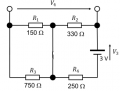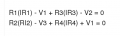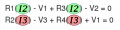# Calculating current and voltage?

#### The Electrician

Joined Oct 9, 2007
2,847
Not true. I think you have too many names for the same variable. Try looking at where the same current flows through several components, then give that current a single name. Now try writing the KVL equations again. I'll bet you have enough equations to solve for the variables. My suggestion for the named currents is here:
View attachment 138669
Did you read the first thing he said in post #1: "How would I go about calculating the branch currents and voltage V4 in this circuit?"

#### RBR1317

Joined Nov 13, 2010
654
Did you read the first thing he said in post #1...
Of course I did. But what point are YOU trying to make (or imply)?

#### The Electrician

Joined Oct 9, 2007
2,847
Of course I did. But what point are YOU trying to make (or imply)?
Oftimes a poster will ask for a nodal solution to a network that, to you and me, cries out for a mesh solution, or for a mesh solution when a nodal solution would be easier. Use of a particular method may be required by the problem. The TS seems to be stuck on finding branch currents, so maybe it's a requirement; we should ask him, or just guide him to a branch analysis: https://www.allaboutcircuits.com/textbook/direct-current/chpt-10/branch-current-method/

#### RBR1317

Joined Nov 13, 2010
654
we should ask him, or just guide him to a branch analysis:
OK - so you just did.

However, the TS was trying to write KVL equations but complained of having too many variables. My response was not for a mesh analysis, but rather geared for how to use fewer variables.

#### The Electrician

Joined Oct 9, 2007
2,847
OK - so you just did.

However, the TS was trying to write KVL equations but complained of having too many variables.
One of the disadvantages of branch analysis is that one usually ends up with more variables than with mesh or nodal analysis. The large number of variables he proposed (and complained of) in post #17 seemed indicative of an attempt at branch analysis, which is consistent with his post #1 request for help in finding branch currents.

That he complains of too many variables may be a good thing. He will surely be appreciative when he graduates from branch analysis to mesh and nodal analysis.

My response was not for a mesh analysis, but rather geared for how to use fewer variables.
The image in your post #20 had me fooled--it looks just like a mesh analysis. The fewer variables feature of your response is also a characteristic of mesh analysis. I hope you can see why I thought you were proposing a mesh analysis.

#### RBR1317

Joined Nov 13, 2010
654
The image in your post #20 had me fooled--it looks just like a mesh analysis.
It's close to mesh analysis; but I was careful to not show any current flowing through the V1 source.

#### shteii01

Joined Feb 19, 2010
4,644
I don't get what you two are arguing about.

In Node Voltage Method I represent branch currents in terms of voltage and resistance (I=V/R, a.k.a. Ohm's Law).
In Mesh Current Method I am actually using the branch currents.

#### TimoG

Joined Nov 2, 2017
17
Not true. I think you have too many names for the same variable. Try looking at where the same current flows through several components, then give that current a single name. Now try writing the KVL equations again. I'll bet you have enough equations to solve for the variables. My suggestion for the named currents is here:
View attachment 138669
OK thanks. That helped a lot.
Is it then reasonable to assume that no current flows through V1 as applying KCL at two nodes gives:
I1 = I2 - I3
I1 = I2 - I3
And therefore either no current flows through V1 or it is indeterminable.

#### The Electrician

Joined Oct 9, 2007
2,847
OK thanks. That helped a lot.
Is it then reasonable to assume that no current flows through V1 as applying KCL at two nodes gives:
I1 = I2 - I3
I1 = I2 - I3
And therefore either no current flows through V1 or it is indeterminable.
Are you required to use a particular method to solve your problem, or can you use any method you please?

#### MrAl

Joined Jun 17, 2014
8,382
Hi,

Here's a suggestion.

The total voltage drop across a source in series with a resistor and no other connection is the same if we swap the source and resistor. For this circuit i think we can swap two sources with two resistors and get a circuit where all three sources have one terminal that is connected to a common node which we can then call ground. We can then simply calculate the two voltages on each side of the required solution voltage and the difference is the solution of that voltage.
So we end up with two voltage dividers where the difference voltage is the solution voltage.

#### TimoG

Joined Nov 2, 2017
17
Are you required to use a particular method to solve your problem, or can you use any method you please?
Any method can be used to solve this problem. I am just confused as to which is the simplest/most effective in this situation.

#### Jony130

Joined Feb 17, 2009
5,241
I am just confused as to which is the simplest/most effective in this situation.
The most efficient way is the method you know the best. So what method you know the best. Or you feel the most comfortable with.

#### RBR1317

Joined Nov 13, 2010
654
Is it then reasonable to assume that no current flows through V1 as applying KCL at two nodes gives:
I1 = I2 - I3
I1 = I2 - I3
If I1 is the current that flows through the V1 source, then the only way for I1 to be zero would be that I2=I3. But solving for I2 & I3 is necessary before finding the answers you seek.

#### The Electrician

Joined Oct 9, 2007
2,847
I would use superposition.

TimoG, if you replace V2 and V3 with short circuits keeping V1, can you calculate the current in each resistor?

After doing that, replace V1 and V2 with short circuits keeping V3, can you calculate the current in each resistor?

After doing that, replace V1 and V3 with short circuits keeping V2, can you calculate the current in each resistor?

Having done those 3 things, add up all the currents and you're problem is solved.

#### TimoG

Joined Nov 2, 2017
17
I would use superposition.

TimoG, if you replace V2 and V3 with short circuits keeping V1, can you calculate the current in each resistor?

After doing that, replace V1 and V2 with short circuits keeping V3, can you calculate the current in each resistor?

After doing that, replace V1 and V3 with short circuits keeping V2, can you calculate the current in each resistor?

Having done those 3 things, add up all the currents and you're problem is solved.
So with V2 and V1 as short circuits, this is the resulting diagram:Where V1 was has formed a short circuit effectively eliminating R1 and R3 from the equation and having the only resistance as R2 and R4 in series. Is this still a valid analysis for the problem?

#### The Electrician

Joined Oct 9, 2007
2,847
So with V2 and V1 as short circuits, this is the resulting diagram:
View attachment 138740

Where V1 was has formed a short circuit effectively eliminating R1 and R3 from the equation and having the only resistance as R2 and R4 in series. Is this still a valid analysis for the problem?
Absolutely. That's what some of the discussion earlier in this thread was about. V1 isolates the two halves of the circuit from each other. Keep going, you're on the right track.

#### RBR1317

Joined Nov 13, 2010
654
Where V1 was has formed a short circuit effectively eliminating R1 and R3 from the equation and having the only resistance as R2 and R4 in series. Is this still a valid analysis for the problem?
Remember these KVL equations you provided back in post #17?Since the same current flows in R1 & R3, you could substitute the named current I2 for IR1 & IR3. Likewise, the same current flows in R2 & R4 so you could substitute the named current I3 for IR2 & IR4. Then you would have a set of two equations in two variables. Also, since these two currents are independent of each other (each has its own equation), each can be solved for independently. What could be simpler than that?#### TimoG

Joined Nov 2, 2017
17
Remember these KVL equations you provided back in post #17?
View attachment 138747
Since the same current flows in R1 & R3, you could substitute the named current I2 for IR1 & IR3. Likewise, the same current flows in R2 & R4 so you could substitute the named current I3 for IR2 & IR4. Then you would have a set of two equations in two variables. Also, since these two currents are independent of each other (each has its own equation), each can be solved for independently. What could be simpler than that?

View attachment 138748
Thank you TheElectrician and RBR1317. I tried both methods and got the same result of I2=3.33mA and I3=3.45mA. This does mean there is current flowing thru V1 of 0.12mA. (in the direction + to - of the battery)
Then for calculating V4 I used the following equation:
V4 = R2(I3) + R1(I2) = 1.64V which seems resonable.

#### The Electrician

Joined Oct 9, 2007
2,847
Once you make the key observation that V1 isolates the effect of each loop on the other, you can proceed to a determination of I2, I3 and thence V4 by inspection.

Clearly the current I2 is the sum of the two voltage sources divided by the sum of the two resistors--> I2 = (V1+V2)/(R1+R3). The voltage across R1 is R1*(V1+V2)/(R1+R3).

Doing the same for I3 you get I3 = (V3-V1)/(R2+R4). The voltage across R2 is R2*(V3-V1)/(R2+R4)

Finally, V4 is the sum of the two--Bada Bing, Bada Boom.